Next: Optimising the cost function Up: Learning algorithm for the Previous: Learning algorithm for the   Contents

## Evaluating the cost function

As before, the general cost function of ensemble learning, as given in Equation (3.11), is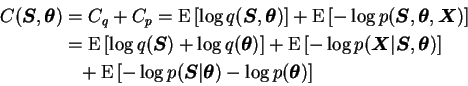(6.25)

where the expectations are taken over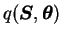. This will be the case for the rest of the section unless stated otherwise.

In the NSSM, all the probability distributions involved are Gaussian so most of the terms will resemble the corresponding ones of the CDHMM. For the parameters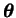,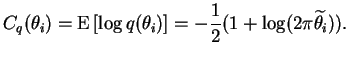(6.26)

The term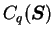is a little more complicated: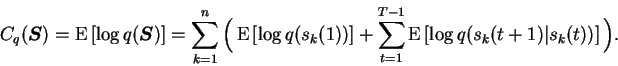(6.27)

The first term reduces to Equation (6.26) but the second term is a little different:The expectation of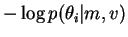has been evaluated in Equation (6.5), so the only remaining terms are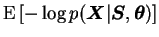and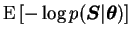. They both involve the nonlinear mappings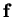and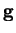, so they cannot be evaluated exactly.

The formulas allowing to approximate the distribution of the outputs of an MLP networkare presented in Appendix B. As a result we get the posterior mean of the outputs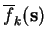and the posterior variance, decomposed as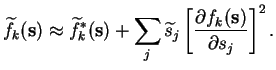(6.28)

With these results the remaining terms of the cost function are relatively easy to evaluate. The likelihood term is a standard Gaussian and yields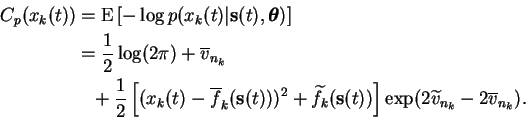(6.29)

The source term is more difficult. The problematic expectation is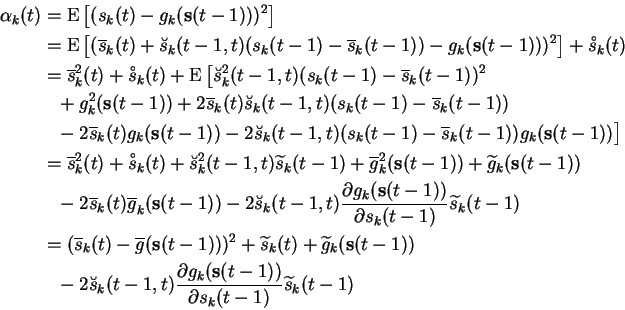(6.30)

where we have used the additional approximationUsing Equation (6.31), the remaining term of the cost function can be written as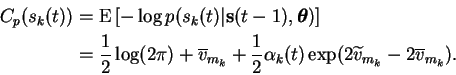(6.31)Next: Optimising the cost function Up: Learning algorithm for the Previous: Learning algorithm for the   Contents
Antti Honkela 2001-05-30